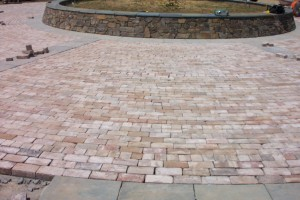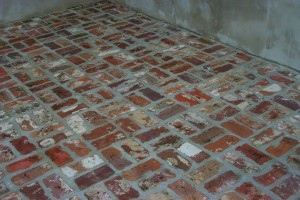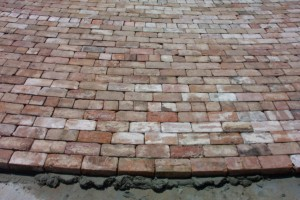###The greatest expense you will incur when you do-it-yourself driveway, walkway or patio project is from the brick pavers. Brick pavers come in numerous styles and sizes and typically cost between \$.50 and \$1.25 per brick, depending on where you purchase them. Next, to the total cost of the brick, the cost of the mortar, the edging, the sand and the gravel is comparatively small.

Step 1: Determine the Square Footage of Work Area

Find out how much square footage you have to cover. Some pavers may measure 3″ by 5″, but don’t count on it. With bricks, especially, much of their charm has to do with the pattern they create. With your tape, measure the width and length of the space to be covered. With odd shapes such as curved areas, the square footage estimation may be rough without resorting to some complex equations. Triangular areas are easy enough. Measure the base and the height of the triangle. No matter the shape of the triangle, those lines will be perpendicular to one another. Multiply them to get the area.

Step 2: Decide Which Size Brick Paver You Will Use

Brick pavers come in numerous sizes. For simplicity’s sake, assume you will use the 1 7/8 x5 inch mortarless paver. The depth is unimportant for this calculation. The length and width and whether you use mortar will affect the price per square foot.Step 3: Sketch the Pattern

Assuming you will lay out the mortarless bricks in a basket weave pattern consisting of alternating pairs of horizontally-placed and vertically-placed pavers, you have to fit that pattern into your work area. The pairs will be placed side by side width-wise. One pair of each side by side is a total of 8 inches up and down by 16 inches across.

Step 4: Calculate Number of Bricks per Row

Length multiplied by width, that is your total square feet. 12 inches to a foot, 6×12=72 total inches wide. 30×12=360 total inches in length. One set of pairs (2 vertical bricks next to 2 horizontal bricks) equals 16 inches wide, which allows you to place 4 full sets of 4 bricks each across with 8 inches to spare. You can finish off one row with either a vertical or a horizontal pair that perfectly fills that 8-inch space. Thus, one row requires 18 bricks.

Step 5: Calculate Bricks per Column

Knowing there is a length of 360 inches and that a set of pairs lengthwise is also 16 inches, you can fit 22 full sets in one column with 8 inches left over. With 4 bricks per set plus 2 additional bricks, that equals 90 bricks per column.Step 6: Calculate Total Bricks and Price per Square Foot

18 bricks per row multiplied by 45 (22.5, the number of sets of 4 bricks, multiplied by 2 because each row is only 2 bricks wide) rows equals 810 total bricks. Assuming you’ve decided to use the paver that costs \$.50 each, that makes a total of \$405 for the pavers. Divide that number by the total square footage, 180, and you get \$2.25 per square foot.

Your calculations will get more complex if you’re placing mortar in the joints or if there are odd shapes to factor in. This estimation, however simplistic, breaks down the steps similar to your custom project. The variables are the number of bricks per row and column, the price per brick and the total square footage.